# I.E. Irodov Solutions on Wave Properties of Particles. Schrodinger Equation

The Schrodinger equation defines the form of the possibility waves (or wave functions) that govern the motion of small particles, and it specifies how these waves are altered by external influences. IE IRODOV Physics Solutions help students to gain a complete understanding and knowledge of this topic. It covers almost all varieties of questions based on the theoretical concepts and deals with all the problems related to wave-particle and its nature.

The problems and solutions are as per today’s syllabus and academic curriculum. This aids the students in simple studying and cracking the upcoming examinations in an easier manner. The questions and answers go hand in hand with the theoretical concepts and connect with the day to day practical applications. This makes the students more confident about the concerned topic.

### I.E. Irodov Solutions on Wave Properties of Particles. Schrodinger Equation

1. Calculate the de Broglie wavelengths of an electron, proton, and uranium atom, all having the same kinetic energy 100 eV.

Solution:

1. The kinetic energy is non relativistic in all three cases. Now

$\lambda =\frac{2\pi h}{P}=\frac{2\pi h}{\sqrt{2mT}}$

On using T= 1.602 X 10-17Joules we get

λe = 122.6 pm

λp = 2.86 pm

$\lambda_{p} =\frac{\lambda _{v}}{\sqrt{238}}=0.815 pm$

A mass number of 238 for the Uranium nucleus

2. What amount of energy should be added to an electron to reduce its de Broglie wavelength from 100 to 50 pm?

Solution:

1. From the equation

$\lambda =\frac{2\pi h}{P}=\frac{2\pi h}{\sqrt{2mT}}$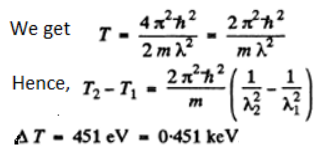3. A neutron with kinetic energy T = 25 eV strikes a stationary deuteron (heavy hydrogen nucleus). Find the de Broglie wavelengths of both particles in the frame of their centre of inertia.

Solution:

1. Now will use M0 = 2Mn. The CM is moving with velocity with respect to Lab frame. In the CM frame the velocity of neutron is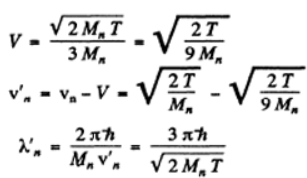On substitution we get λ’n= 8.6pm

Since the momenta are equal in the CM frame the de Broglie wavelengths will also be equal. If we do not assume Md ≅ 2Mn

$\lambda'_{n} =\frac{2\pi h(1+\frac{M_{n}}{M_{d}})}{\sqrt{2M_{n}T}}$

4. Two identical non-relativistic particles move at right angles to each other, possessing de Broglie wavelengths λ1 and λ2. Find the de Broglie wavelength of each particle in the frame of their centre of inertia.

Solution:

1. If $\vec{P_{1}}$, $\vec{P_{2}}$ are the momenta of the two particles then their momenta in the CM frame will be

$\frac{\vec{P_{1}-\vec{P_{2}}}}{2}$ as the particles are identical.

Hence their de Broglie wavelength will be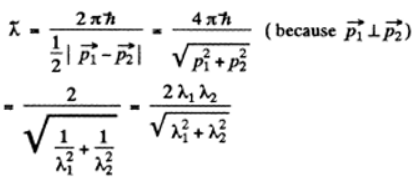5. Find the de Broglie wavelength of hydrogen molecules, which corresponds to their most probable velocity at room temperature.

Solution:

1. In thermodynamic equilibrium, Maxwell’s velocity distribution law holds

d N(v) = ϕ(v) dv = Av2e-mv^2/2kT dv

ϕ(v) is minimum when

ϕ’(v) = ϕ(v)[2/v - mv/kT] = 0

The defines the most probable velocity

Vpt = √(2kT)/m

The de Broglie wavelength of H molecules with the most probable velocity is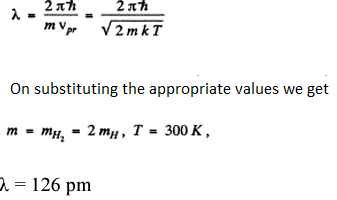6. Calculate the most probable de Broglie wavelength of hydrogen molecules being in thermodynamic equilibrium at room temperature.

Solution:

1. To find the most probable de Broglie wavelength of a gas in thermodynamic equilibrium we determine the distribution is λ corresponding to Maxwellian velocity distribution.

It is given by the equation

$\varphi (\lambda )d\lambda =-\phi (v)dv$

where - sign takes account of the fact that λ decreaes as v increases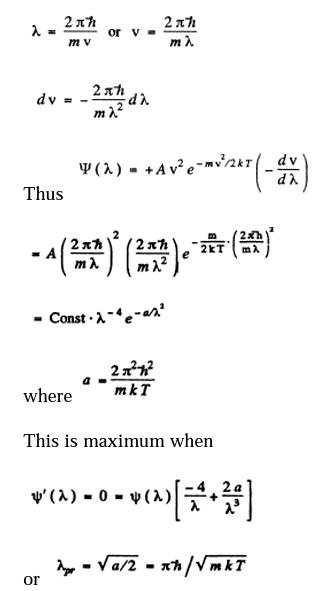Using the result of the previous problem it is

$\lambda _{pr}=\frac{\sqrt{126}}{2}=89.1pm$

7. Derive the expression for a de Broglie wavelength λ of a relativistic particle moving with kinetic energy T. At what values of T does the error in determining λ using the non-relativistic formula not exceed 1% for an electron and a proton?

Solution:

1. For a relativistic particle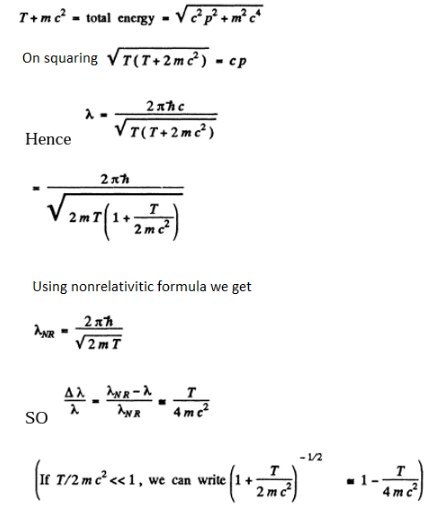Thus,

$T\leq \frac{4mc^{2}\Delta \lambda}{\lambda }$ if the error is less than ∆λ

For electron the error is not more than 1 % if

T≤4 x 0.511 x .01MeV

≤ 20.4KeV

For a proton, the error is not more than 1 % if

T ≤ 4 X 938 X 0.01 MeV

i.e.T ≤ 37.5 MeV.

8. At what value of kinetic energy is the de Broglie wavelength of an electron equal to its Compton wavelength?

Solution:

1. The de Broglie wavelength is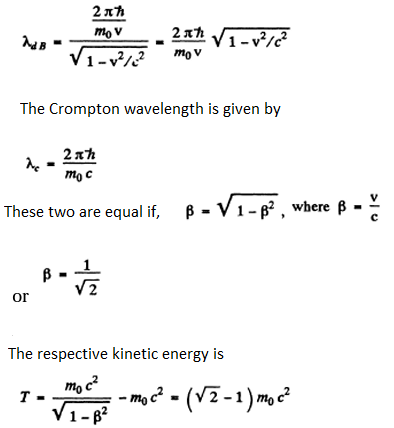Here m0 is the rest mass of the particle (here an electron).

9. Find the de Broglie wavelength of relativistic electrons reaching the anticathode of an X-ray tube if the short wavelength limit of the continuous X-ray spectrum is equal to λsh= 10.0 pm?

Solution:

1. For relativistic electrons, the formula for the short wavelength limit of X - rays will be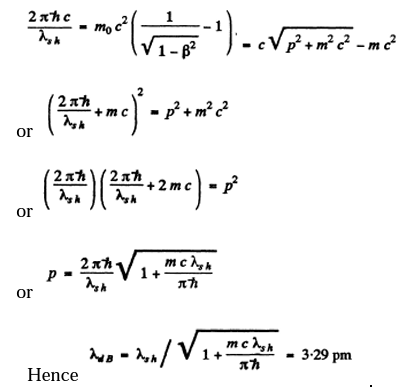10. A parallel stream of monoenergetic electrons falls normally on a diaphragm with a narrow square slit of width b = 1.0 μm. Find the velocity of the electrons if the width of the central diffraction maximum formed on a screen located at a distance l = 50 cm from the slit is equal to Δx = 0.36 mm.

Solution:

1. The first minimum in a Fraunhofer diffraction is given by (b is the width of the slit)

b sinθ=λ

Now,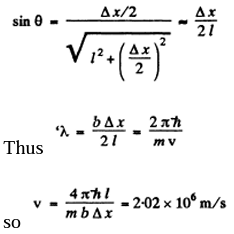11. A parallel stream of electrons accelerated by a potential difference V = 25 V falls normally on a diaphragm with two narrow slits separated by a distance d = 50μm. Calculate the distance between neighbouring maxima of the diffraction pattern on a screen located at a distance l = 100 cm from the slits.

Solution:

1. From the Young’s slit formula

$\Delta x=\frac{l\lambda }{d}=\frac{l}{d}\frac{2\pi h}{\sqrt{2meV}}$

On substituting the given values we get

∆x= 4.90 pm

12. A narrow stream of monoenergetic electrons falls at an angle of incidence θ = 30° on the natural facet of an aluminium single crystal. The distance between the neighbouring crystal planes parallel to that facet is equal to d = 0.20 nm. The maximum mirror reflection is observed at a certain accelerating voltage V0 . Find Vo, if the next maximum mirror reflection is known to be observed when the accelerating voltage is increased η = 2.25 times.

Solution:

1. For the first case, from Bragg’s law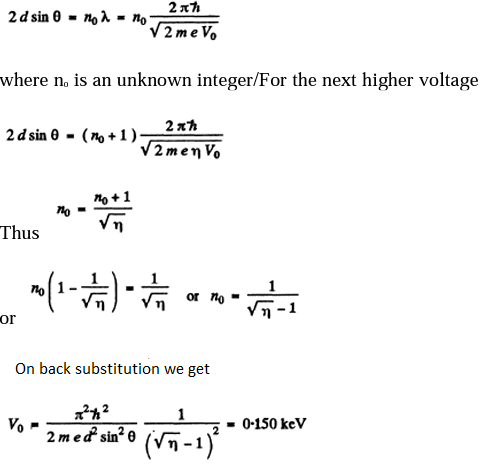[Note: In the Bragg’s formula, θ is the glancing angle and not the angle of incidence. We have obtained the correct result by taking θ to be the glancing angle. If θ is the angle of incidence, then the glancing angle will be 90 - θ. Then the final answer will be smaller by a factor tan2θ=⅓]

13. A narrow beam of monoenergetic electrons falls normally on the surface of a Ni single crystal. The reflection maximum of fourth-order is observed in the direction forming an angle θ = 55° with the normal to the surface at the energy of the electrons equal to T = 180 eV. Calculate the corresponding value of the interplanar distance.

Solution:

1. Path difference is

d+d cosθ= 2d cos2θ/2

Thus for reflection maximum of the kth order

$2d\cos^{2}\frac{\Theta }{2}=k\lambda =k\frac{2\pi h}{\sqrt{2mT}}$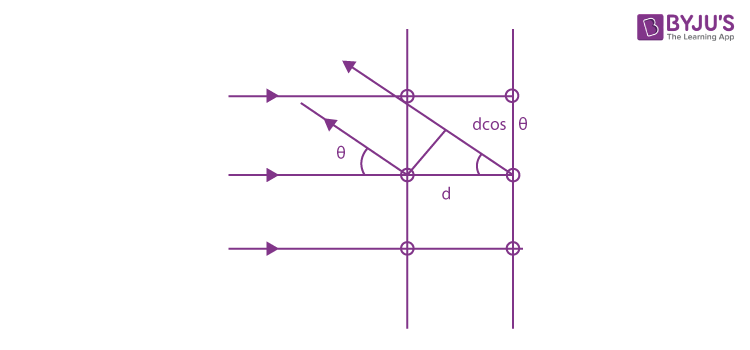Hence,

$d=\frac{k\pi h}{\sqrt{2mT}}\sec^{2}\frac{\Theta }{2}$

substitution with k = 4

We get

d = 0.232

14. A narrow stream of electrons with kinetic energy T = 10 keV passes through a polycrystalline aluminium foil, forming a system of diffraction fringes on a screen. Calculate the interplanar distance corresponding to the reflection of third-order from a certain system of crystal planes if it is responsible for a diffraction ring of diameter D = 3.20 cm. The distance between the foil and the screen is l = 10.0 cm.

Solution:

1. The glancing angle is obtained from

$\tan2\Theta =\frac{D}{2l}$

where D = rays (5.156) diameter of the ring, l = distance from the foil to the screen.

Then for the third-order Bragg reflection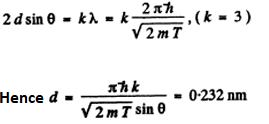15. A stream of electrons accelerated by a potential difference V falls on the surface of a metal whose inner potential is V1 = 15 V. Find:

(a) the refractive index of the metal for the electrons accelerated by a potential difference V = 150 V;

(b) the values of the ratio V/Vt at which the refractive index differs from unity by not more than η = 1.0%.

Solution:

1. Inside the metal, there is a negative potential energy of - eVi. (This potential energy prevents electrons from leaking out and can be measured in photoelectric effect etc.) An electron whose K.E. is eV outside the metal will find its K.E. increased to e (V + Vi) in the metal. Then

(a) de Broglie wavelength in the metal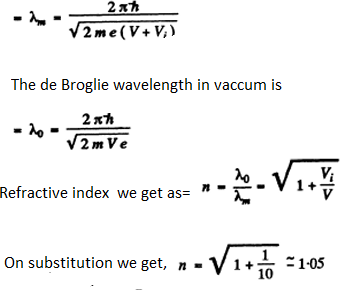(b)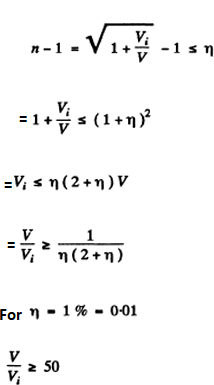16. A particle of mass m is located in a unidimensional square potential well with infinitely high walls. The width of the well is equal to l. Find the permitted values of energy of the particle taking into account that only those states of the particle's motion are realized for which the whole number of de Broglie half within the given well.

Solution:

1. The energy inside the well is all kinetic if energy is measured from the value inside. We require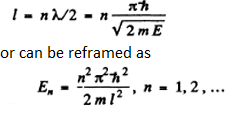17. Describe the Bohr quantum conditions in terms of the wave theory: demonstrate that an electron in a hydrogen atom can move only along those round orbits which accommodate a whole number of de Broglie waves.

Solution:

1. The Bohr condition

$\phi pdx=\phi \frac{2\pi h}{\lambda } dx=2\pi nh$

For the case when λ is constant (for example in circular orbits)

This means 2nr = nλ

Here, r is the radius of the circular orbit.

18. Estimate the minimum errors in determining the velocity of an electron, a proton, and a ball of mass of 1 mg if the coordinates of the particles and of the centre of the ball are known with an uncertainty of 1µm.

Solution:

1. From the uncertainty principle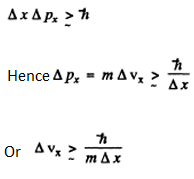For an electron, this means an uncertainty in velocity of 116 m/s if Δx= 10-6m= 1μm

For a proton, ΔVx = 6.3 cm/s

For a ball, ΔVx = 1 x 10-20cm/s

19. Employing the uncertainty principle, evaluate the indeterminacy of the velocity of an electron in a hydrogen atom if the size of the atom is assumed to be l = 0.10 nm. Compare the obtained magnitude with the velocity of an electron in the first Bohr orbit of the given atom.

Solution:

1. As in the previous problem

The actual velocity v1 has been calculated in problem 6.21.

It is v, = 2.21 x 106 m/s

Thus, ∆v∼v1 (They are of the same order of magnitude)

20. Show that for the particle whose coordinate uncertainty is Δx = λ/2π, where λ is its de Broglie wavelength, the velocity uncertainty is of the same order of magnitude as the particle's velocity itself.

Solution:

1.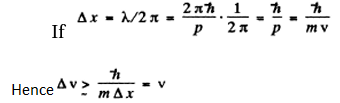Thus Δv is of the same order as v